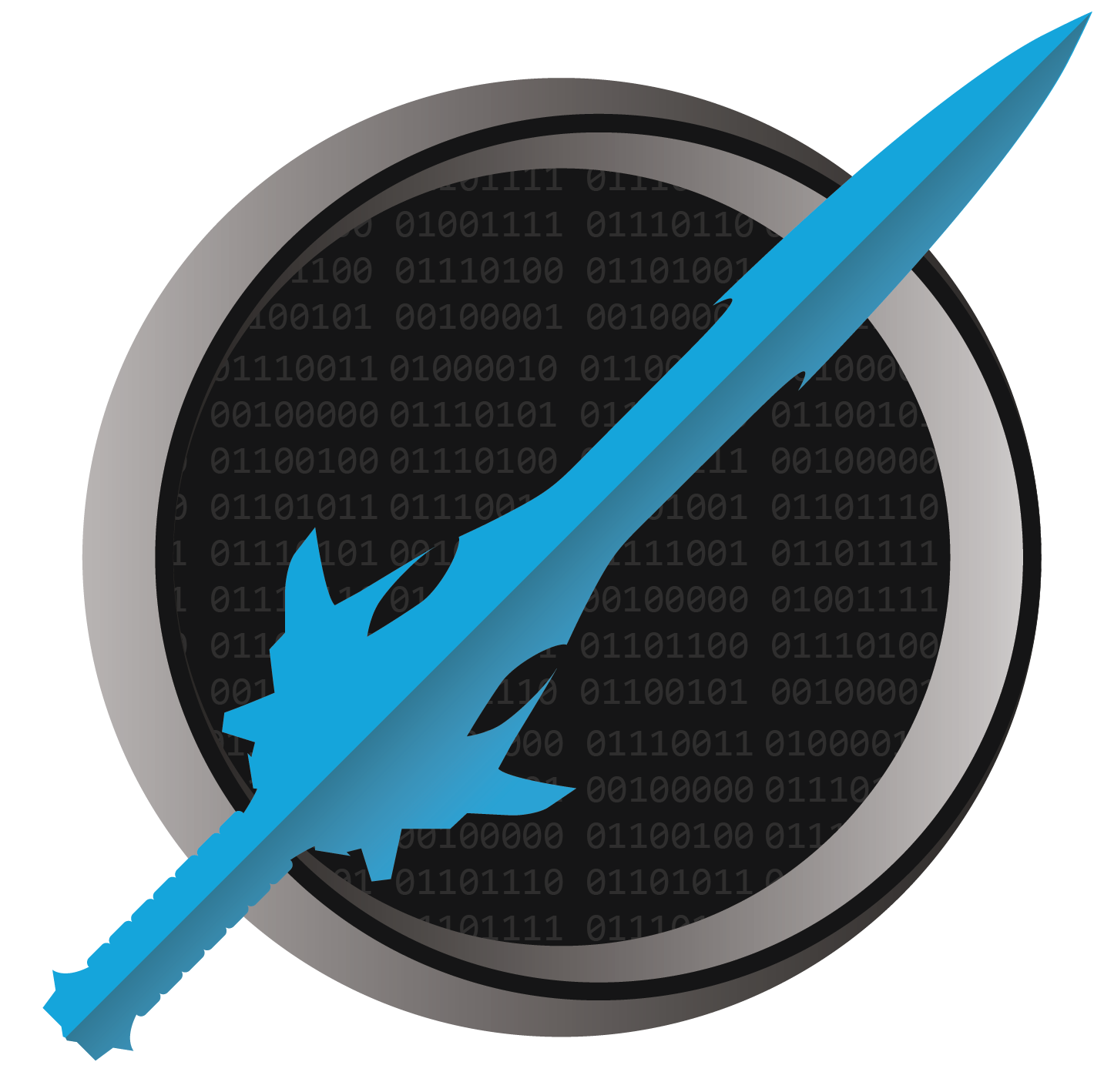It's dangerous to code alone! Take this.

# Many Random Words

The following is one possible solution to this challenge.

``````using System;

while (true)
{
Console.Write("Enter a word to randomly regenerate: ");
HandleWord(word);
}

{
DateTime start = DateTime.Now;
int attempts = await RandomlyRecreateAsync(word);
Console.WriteLine(\$"The word {word} took {attempts} attempts.");
TimeSpan elapsed = DateTime.Now - start;
Console.WriteLine(elapsed);
}

int RandomlyRecreate(string word)
{
Random random = new Random();

string generated;
int attempts = 0;
do
{
attempts++;
generated = "";
for (int letter = 0; letter < word.Length; letter++)
generated += (char)('a' + random.Next(26));
} while (generated != word);

return attempts;
}